Mathematics
Similar Polygons
18.6k views

 1 Introduction 2 Similar Polygons 3 Similar Quadrilaterals 4 Similar Rectangles 5 Summary 6 FAQs

03 November 2020

## Introduction

Sizes and shapes are the backbones of geometry. One of the most encountered shapes in geometry is polygons. The Greek word ‘Polygon’ consists of Poly meaning ‘many’ and gon meaning ‘angle.’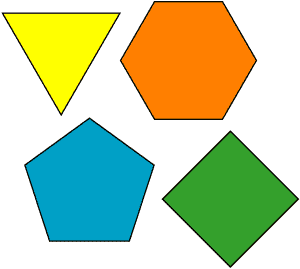Polygons are two-dimensional shapes composed of straight lines. They are said to have a ‘closed shape’ as all the lines are connected. In this article, we will discuss the concept of similarity in Polygons.

## Similar Polygons - PDF

If you ever want to read it again as many times as you want, here is a downloadable PDF to explore more.

## Similar Polygons

First, let us get clear with what ‘similar’ means. Two things are called similar when they both have a lot of the same properties but still may not be identical. The same can be said about polygons.

### Congruent polygons

As you might have studied, Congruent shapes are the shapes that are an exact match. Congruent polygons have the same size, and they are a perfect match as all corresponding parts are congruent or equal.

### Similar polygons definition

On the other hand, In Similar polygons, the corresponding angles are congruent, but the corresponding sides are proportional. So, similar polygons have the same shape, whereas their sizes are different. There would be certain uniform ratios in similar polygons.

### Properties of similar polygons

There are two crucial properties of similar polygons:

• The corresponding angles are equal/congruent. (Both interior and exterior angles are the same)
• The ratio of the corresponding sides is the same for all sides. Hence, the perimeters are different.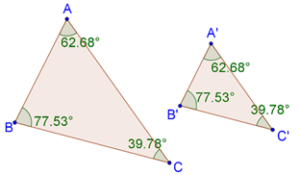The above image shows two similar polygons(triangles), ABC, and A’B’C’. We can see that corresponding angles are equal.

$<A=<A', <B=<B',<c=<C'$

The corresponding sides have the same ratios.

$\frac{AB}{A'B'}=\frac{BC}{B'C'}=\frac{CA}{C'A'}$

Quadrilaterals are polygons that have four sides. The sum of the interior angles of a quadrilateral is 360 degrees. Two quadrilaterals are similar quadrilaterals when the three corresponding angles are the same( the fourth angles automatically become the same as the interior angle sum is 360 degrees), and two adjacent sides have equal ratios.

### Are all squares similar?

Let us discuss the similarity of squares. According to the similarity of quadrilaterals, the corresponding angles of similar quadrilaterals should be equal. We know that all angles are 90 degrees in the square, so all the corresponding angles of any two squares will be the same.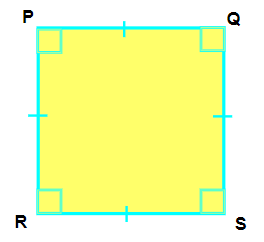All sides of a square are equal. If let’s say, square1 has a side length equal to ‘a’ and square2 has a side length equal to ‘b’, then all the corresponding sides' ratios will be the same and equivalent to a/b.

Hence, all squares are similar squares.

### Are all rhombuses similar?

In a Rhombus, all the sides are equal. So, just like squares, rhombuses satisfy the condition of the ratio of corresponding sides being equal.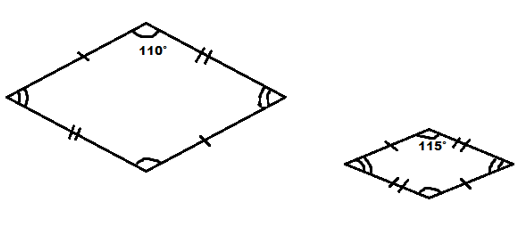In a Rhombus, the opposite sides are parallel, and hence the opposite angles are equal. But the value of those angles can be anything. So, it can very much happen that two rhombuses have different angles. Hence, all rhombuses are not similar.

## Similar Rectangles

Two rectangles are similar when the corresponding adjacent sides have the same ratio. We do not need to check the angles as all angles in a rectangle are 90 degrees.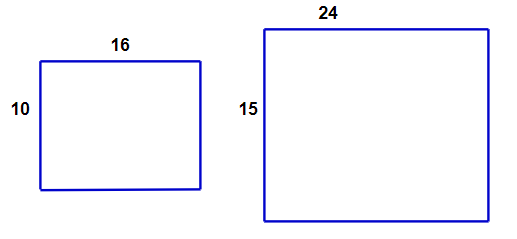In the above image, the ratios of the adjacent side are . Hence, these are similar rectangles.

### Are all rectangles similar?

No, all rectangles are not similar rectangles. The ratio of the corresponding adjacent sides may be different. For example, let’s take a 1 by 2 rectangle and take another rectangle with dimensions 1 by 4. Here the ratios will not be equal.

$\frac{1}{1}\ne\frac{4}{2}$

### Congruent rectangles

Two rectangles are called congruent rectangles if the corresponding adjacent sides are equal. It means they should have the same size. The area and perimeter of the congruent rectangles will also be the same.

## Summary

Similarity and congruency are some important concepts of geometry. A solid understanding of these topics helps in building a good foundation in geometry. This article discussed the concepts of similarity in polygons looking at some specific cases of similar quadrilaterals like similar squares, similar rectangles, and similar rhombuses.

No, rectangles are not always congruent when they have the same perimeter. The ratio of lengths of corresponding sides may be different even when the perimeter is the same. For example, a rectangle of 5 by 4 and another rectangle of 6 by 3 has the same perimeter(equal to 18), but the corresponding sides' ratios are different $$\frac{5}{6}\ne\frac{4}{3}$$.